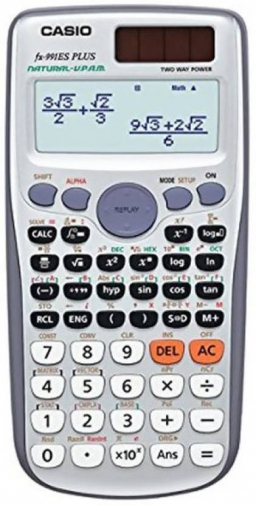# Calculate: 25551

Calculate:
a) 17 + (-23) - (-42) =
b) - 32 - (+12) + (+13) =
c) 6.7 - (+ 2.5) - (- 8.2) =
d) - 0.56 - 4.38 + 6.78 =

a =  36
b =  -31
c =  12.4
d =  1.84

### Step-by-step explanation:Did you find an error or inaccuracy? Feel free to write us. Thank you!